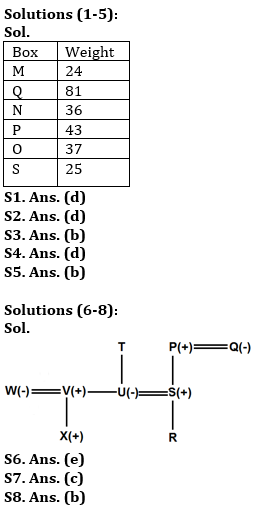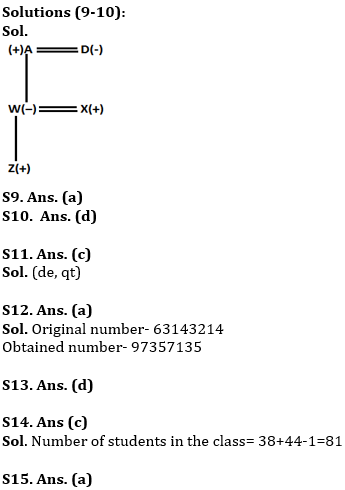Latest Banking jobs   »   Reasoning Ability Quiz For IBPS RRB...

# Reasoning Ability Quiz For IBPS RRB PO, Clerk Prelims 2021- 28th July

Six boxes M, N, O, P, Q, and S are placed one above another, also they are of different weights (in kg.) i.e. 24, 25, 36, 37, 43, and 81(but not necessarily in the same order). Box P’s weight is not a lowest among all the boxes. M is placed either top or bottom. The weight of the box which is placed at bottom is 25kg. Only one box is placed between P and S. Box Q is not placed just above and below P. Box O is placed below Q but not just below Q. The weight of the box which is placed just above N is a perfect square of an odd number. Box O’s weight is a prime number. Box N is not placed above M, whose weight is not a perfect square. Only 2 boxes are placed between M and P, whose weight is a prime number. Box P is not third heaviest box.

Q1. What is the weight of Box O?
(a) 24
(b) 25
(c) 36
(d) 37
(d) None of these

Q2. Which of the following box is placed at top?
(a) N
(b) S
(c) O
(d) M
(d) None of these

Q3. How many boxes are placed between Q and the box which weight’s is 25?
(a) One
(b) Three
(c) Two
(d) More than three
(d) None of these

Q4. Which of following box is 24kg?
(a) P
(b) N
(c) Q
(d) M
(e) None of these

Q5. What is the weight of the box which is placed immediate below N?
(a) 36
(b) 43
(c) 24
(d) 81
(e) None of these

Directions (6-8): Study the information carefully and answer the questions given below.
There are nine members in a family, in which only three married couples. There are three generation in this family. Q is the wife of P. P is the grandfather of R. Q has only one son who is married to T’s child. T has only two children one son and one daughter. X is grandson of T. S is brother in law of son of T. U and V are children of T. W is married to the son of T. X is nephew of U and he is W’s child. U is a married woman.

Q6. If R is married to Y, then how is Y related to S?
(a) Son
(b) Daughter
(c) Son in law
(d) Daughter in law
(e) Can’t be determine

Q7. How is S related to T?
(a) Son
(b) Daughter
(c) Son in law
(d) Daughter in law
(e) Husband

Q8. How is Q related to R?
(a) Grandfather
(b) Grandmother
(c) Uncle
(d) Aunt
(e) Either (c) or (d)

Directions (9-10): Study the following information carefully and answer the given questions:
In a family of five members, there are two married couples in the family. A is father in law of X, who is married with W. Z is the son of W. D who is a married woman has only one daughter.

Q9. How is A related with Z?
(a) Grand Father
(b) Grand Mother
(c) Father in law
(d) Daughter in law
(e) Grandson

Q10. Who is the son in law of Z’s grandmother?
(a) A
(b) D
(c) W
(d) X
(e) None of these

Q11. How many pairs of letters are there in the word “Adequate” each of which have as many letters between them in the word as they have between them in the English alphabetical series?
(a) Three
(b) One
(c) Two
(d) More than three
(e) None

Q12. If in the number 63143214, addition of 1 after multiplying by 2 in each of the odd digit and subtract 3 after multiplying by 2 in each of the even digit then, How many digits are repeating in new number ?
(a) Three
(b) One
(c) None
(d) Two
(e) Four

Q13. Which of the following elements should come in a place of ‘?’
JQ4 HS9 FU16 ?
(a) EV36
(b) PK 81
(c) IR 100
(d) DW25
(e) LO 49

Q14. Arun’s ranked 38th from the top and 44th from the bottom in a class. How many students are there in the class?
(a) 82
(b) 77
(c) 81
(d) 79
(e) None of these

Q15. If in the number 867351429, all digits are arranged in increasing order from right to left than how many digit remain same place in the new arrangement?
(a) Three
(b) Four
(c) None
(d) Two
(e) One

Practice More Questions of Reasoning for Competitive Exams:

###### Study Plan for IBPS RRB PO/Clerk Prelims 2021

Solutions#### Congratulations!